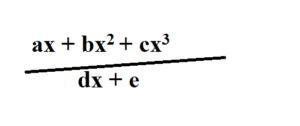# Tag: AMath Polynomials## Summary Notes for Polynomials and Partial Fractions

Here are summary for polynomials (factor, remainder theorem, and solving cubic equations): Remainder Theorem: For a polynomial P(x), when P(x) is divided by (x -b), the remainder…## What you need to know for Polynomials and Partial Fractions tested in O Level Additional Mathematics

Polynomials (including factor and remainder theorem and cubic equations) and partial fractions are easy to score for O Level Additional Mathematics, if you know the…

error: Content is protected !!